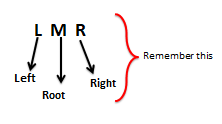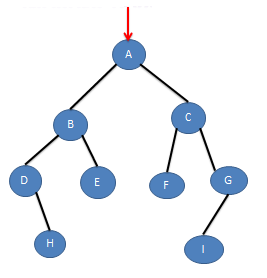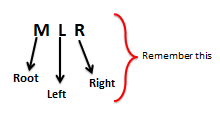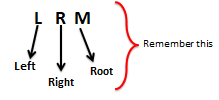# Traversing a Binary Tree in Data Structure – InOrder, PreOrder and PostOrder

First of all you should know What Traversing Mean.
So It Simply means Travelling or Visiting all the nodes of the tree once.

So you have 3 ways , how can travel in a Binary Tree. They are

• Inorder
• Preorder
• Postorder
• ## InOrder

For Inorder traversal remember that the Root node is come in-between.Here keep in mind that you go to the left node(L) first than Read the root node(M) and then finally go to the right node(R).

Lets take an example, and we will do Inorder traversingBy keeping in mind the LMR formula we can write following Algo for Inorder Traversal.
```Inorder(root) 1. If(root == null)    Exit 2. Inorder(left child of root) //Recursive func; L 3. Visit (root) // M 4. Inorder(right child of root) //Recursive func; R ```

## PreOrder

For Preorder traversal remember that the Root node comes before left and right.Here keep in mind that you visit the root node(M) first than go to the left node(L) and then finally go to the right node(R).

Lets take an example, and we will do Preorder traversingBy keeping in mind the MLR formula we can write following Algo for Preorder Traversal.
```Preorder(root) 1. If(root == null)    Exit 2. Visit (root) // M 3. Preorder(left child of root) //Recursive func; L 4. Preorder(right child of root) //Recursive func; R```

## PostOrder

For Postorder traversal remember that the Root node comes after left and right.Here keep in mind that you go to the left node(L) first than go to the right node(R) and then finally visit the root node(M).

Lets take an example, and we will do Postorder traversingBy keeping in mind the LRM formula we can write following Algo for Postorder Traversal.
```Postorder(root) 1. If(root == null)    Exit 2. Postorder(left child of root) //Recursive func; L 3. Postorder(right child of root) //Recursive func; R 4. Visit (root) // M```

## One Reply to “Traversing a Binary Tree in Data Structure – InOrder, PreOrder and PostOrder”

1.Madhu says:

Very useful